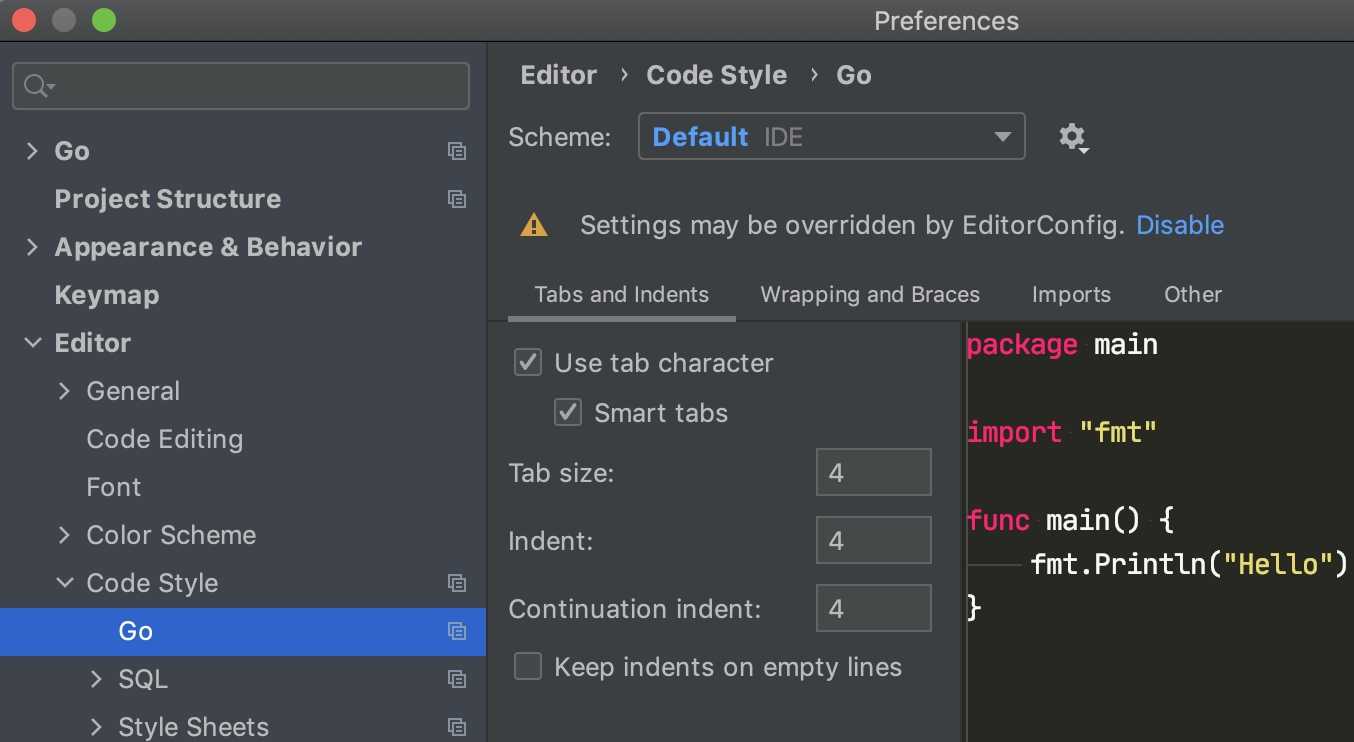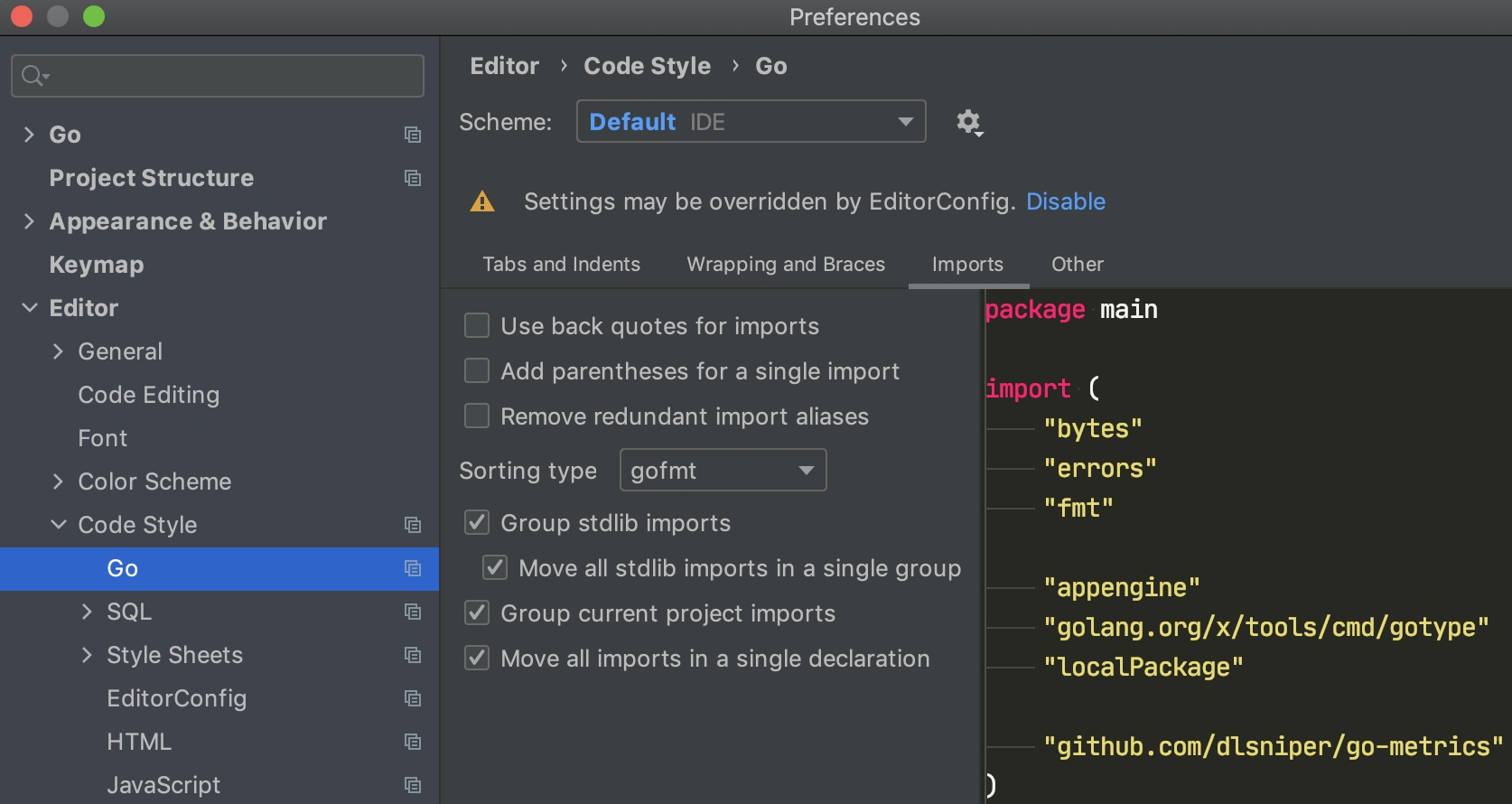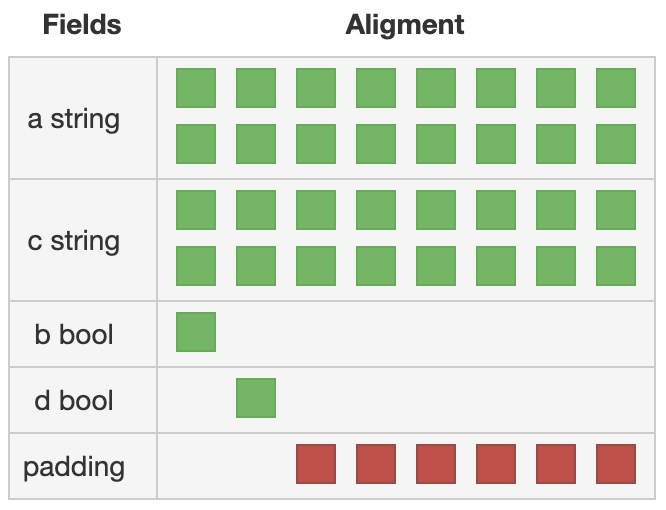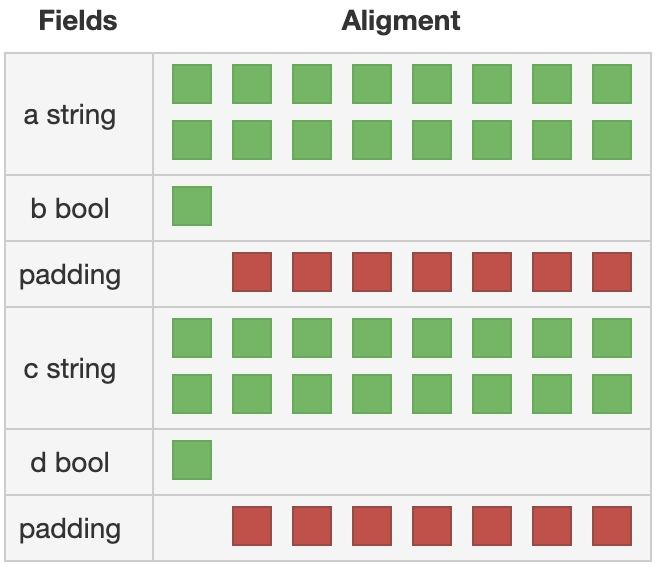GitHub: shockerli/go-code-guide

## 命名规则

• 站在调用者的角度，包不是给你自己用的
• 简洁、且见名知义
• 采用通用、大众熟知的缩写命名。比如`buf`而不是`bufio`
• 如果缩写的名字会产生歧义，则放弃或换个

### 包名

• 包名与目录名一致

如果一个目录下同时出现多个 `package`，则编译失败：

 ``````1 `````` ``````found packages pkg (a.go) and pb (b.go) in XXX ``````
• 大多数使用命名导入的情况下，不需要重命名

少让调用者去起别名，除非名字太烂

• 全部小写，没有下划线、大写。错误示例`MyPackage``my_package``myPackage`

• 不用复数。例如`net/url`，而不是`net/urls`

• 不用信息量不足的名字。错误示例`common``lib``util`

### 导入包

• 如果程序包名称与导入路径的最后一个元素不匹配，则必须使用导入别名
 ``````1 2 3 4 `````` ``````import ( client "example.com/client-go" trace "example.com/trace/v2" ) ``````
• 在所有其他情况下，除非导入之间有直接冲突，否则应避免导入别名
 ``````1 2 3 4 `````` ``````import ( "net/http/pprof" gpprof "github.com/google/pprof" ) ``````
• 如遇重名，请保留标准包而别名自定义或第三方包

• 在非测试文件（`*_test.go`）中，禁止使用 `.` 来简化导入包的对象调用

• 禁止使用相对路径导入（`./subpackage`），所有导入路径必须符合 `go get` 标准

### 驼峰命名法

 ``````1 `````` ``````const ROLE_NAME = 10 ``````

Good:

 ``````1 `````` ``````const RoleName = 10 ``````

### 常量

• 如果是枚举类型的常量，需要先创建相应类型
 ``````1 2 3 4 5 6 `````` ``````type Scheme string const ( Http Scheme = "http" Https Scheme = "https" ) ``````
• 如果模块的功能较为复杂、常量名称容易混淆的情况下，为了更好地区分枚举类型，可以使用完整的前缀
 ``````1 2 3 4 5 6 `````` ``````type Symbol string const ( SymbolAdd Symbol = "+" SymbolSub Symbol = "-" ) ``````

### 变量

• 在相对简单的环境（对象数量少、针对性强）中，可以将一些名称由完整单词简写为单个字母

• `user` 可以简写为 `u`
• `userId` 可以简写 `uid`
• 若变量类型为 `bool` 类型，则名称应以 `Has``Is``Can``Allow` 开头

 ``````1 2 3 4 `````` ``````var isExist bool var hasConflict bool var canManage bool var allowGitHook bool ``````

### URL

• URL 命名全部小写
• 用正斜杠 `/` 表明层级关系
• 使用连字符 `-` 来提高长路径中名称的可读性
• 不得在 URL 中使用下划线 `_`
• URL 结尾不应包含正斜杠 `/`
• 文件扩展名不应包含在 URL 中
• URL 需见名知意，但不可暴露服务器架构

 ``````1 2 3 4 `````` ``````/GetUserInfo /photos_path /My-Folder/my-doc/ /user/user-list ``````

Good:

 ``````1 2 `````` ``````/user/list /user/operator-logs ``````

### 函数/方法名

• 不要画蛇添足

• 长命名并不会使其更具可读性，一份有用的说明文档通常比额外的长名更有价值

 ``````1 `````` ``````once.DoOrWaitUntilDone(f) ``````

Good:

 ``````1 `````` ``````once.Do(f) ``````
• 在 pkg 包中名为 New 的函数会返回一个 pkg.Pkg 类型的值
 ``````1 `````` ``````q := list.New() // q is a *list.List ``````
• 当 pkg 包中某个函数的返回值类型为 pkg.Pkg （或 *pkg.Pkg ）时，函数名应省略类型名
 ``````1 2 `````` ``````start := time.Now() // start is a time.Time t, err := time.Parse(time.Kitchen, "6:06PM") // t is a time.Time ``````
• 当函数返回的值类型为 pkg.T 且 T 不为 Pkg 时，函数名应包含 T 以便让用户代码更易理解
 ``````1 2 `````` ``````ticker := time.NewTicker(d) // ticker is a *time.Ticker timer := time.NewTimer(d) // timer is a *time.Timer ``````
• 获取器/设置器

Go 并不对获取器（getter）和设置器（setter）提供自动支持。针对某个变量或字段，获取器名字无需携带 `Get`，设置器名字以 `Set` 开头。

若你有个名为 owner （小写，未导出）的字段，其获取器应当名为 Owner（大写，可导出）而非 GetOwner。

 ``````1 2 3 4 `````` ``````owner := obj.GetOwner() if owner != user { obj.SettingOwner(user) } ``````

Good:

 ``````1 2 3 4 `````` ``````owner := obj.Owner() if owner != user { obj.SetOwner(user) } ``````
• 若函数或方法为判断类型（返回值主要为 bool 类型），则名称应以 `Has``Is``Can``Allow` 等判断性动词开头
 ``````1 2 3 4 `````` ``````func HasPrefix(name string, prefixes []string) bool { ... } func IsEntry(name string, entries []string) bool { ... } func CanManage(name string) bool { ... } func AllowGitHook() bool { ... } ``````

### 接口名

 ``````1 2 3 `````` ``````type Reader interface { Read(p []byte) (n int, err error) } ``````

### Error

• `Error` 类型的命名以 `Error` 结尾
 ``````1 2 3 `````` ``````type ParseError struct { Line, Col int } ``````
• `Error` 类型的变量，以 `Err`开头
 ``````1 `````` ``````var ErrBadAction = errors.New("somepkg: a bad action was performed") ``````
• 返回类型为 `Error` 的变量缩写采用 `err`
 `````` 1 2 3 4 5 6 7 8 9 10 11 `````` ``````func foo() { res, err := somepkgAction() if err != nil { if err == somepkg.ErrBadAction { } if pe, ok := err.(*somepkg.ParseError); ok { line, col := pe.Line, pe.Col // .... } } } ``````

### 其他

#### 包内容的名字不可以包名开头，因为无需重复包名

`http` 包提供的 HTTP 服务名为 `http.Server` ，而非 `HTTPServer` 。用户代码通过 `http.Server` 引用该类型，因此没有歧义。

## 名词缩写表

`ctx` `Context` 或相关，比如 `gin.Context`

## 分号

Go 其实也是用分号（`;`）来结束语句，但 Go 与 JavaScript 一样不建议给单一语句末尾加分号，因为编译器会自动加分号。

 ``````1 `````` ``````go func() { for { dst <- <-src } }() ``````

 ``````1 2 3 `````` ``````if err := f(); err != nil { g() } ``````

 ``````1 2 3 4 `````` ``````if i < f() // 报错 { // 报错 g() } ``````

## 文档

`README`、项目文档、接口文档等，中文文档的排版参考：中文文案排版指北

## 注释

• 所有导出对象必须注释说明其用途，非导出对象根据情况进行注释

• 如果对象可数且无明确指定数量的情况下，一律使用单数形式和一般进行时描述，否则使用复数形式

• 包、函数、方法和类型的注释说明都是一个完整的句子

• 句子类型的注释首字母均需大写，短语类型的注释首字母需小写

• 注释的单行长度不能超过 80 个字符，超过请强制换行

• 可导出对象的注释，必须以对象的名称作为开头

 ``````1 2 3 4 5 `````` ``````// FileInfo is the interface that describes a file and is returned by Stat and Lstat type FileInfo interface { ... // HasPrefix returns true if name has any string in given slice as prefix func HasPrefix(name string, prefixes []string) bool { ... ``````

### 单行注释&多行注释

• 两种注释风格，单行注释 `//`，多行注释 `/* ... */`

• 多行注释仅用于包级别的文档注释，除此之外请用单行注释。包注释一般放置到 `doc.go` 文件，且该文件仅包含文档注释内容

• 单行注释符号与内容之间，请用一个空格隔开

 ``````1 `````` ``````//Comments ``````

Good:

 ``````1 `````` ``````// Comments ``````

GoLand 可设置自动格式化：

`Preferences > Editor > Code Style > Go > Other` 勾选上 `Add leading space to comments`

### 包注释

• 包级别的注释就是对包的介绍，只需在同个包的任一源文件中说明即可有效

• 对于 `main` 包，一般只有一行简短的注释用以说明包的用途，且以项目名称开头

 ``````1 2 `````` ``````// Write project description package main ``````
• 对于一个复杂项目的子包，一般情况下不需要包级别注释，除非是代表某个特定功能的模块

• 对于简单的非 `main` 包，也可用一行注释概括

• 对于相对功能复杂的非 `main` 包，一般都会增加一些使用示例或基本说明，且以 `Package <name>` 开头

 ``````1 2 3 4 5 `````` ``````/* Package http provides HTTP client and server implementations. ... */ package http ``````
• 特别复杂的包说明，可单独创建 `doc.go` 文件来加以说明

### 函数与方法

• 如果一句话不足以说明全部问题，则可换行继续进行更加细致的描述
 ``````1 2 `````` ``````// Copy copies file from source to target path. // It returns false and error when error occurs in underlying function calls. ``````
• 若函数或方法为判断类型（返回值主要为 `bool` 类型），则以 `<name> returns true if` 开头
 ``````1 2 `````` ``````// HasPrefix returns true if name has any string in given slice as prefix. func HasPrefix(name string, prefixes []string) bool { ... ``````

### 结构、接口及其它类型

• 类型的定义一般都以单数形式描述：
 ``````1 2 `````` ``````// Request represents a request to run a command. type Request struct { ... ``````
• 如果为接口，则一般以以下形式描述：
 ``````1 2 `````` ``````// FileInfo is the interface that describes a file and is returned by Stat and Lstat. type FileInfo interface { ... ``````
• 如果结构体属性较多，需对属性添加注释
 ``````1 2 3 4 5 6 `````` ``````// Var variable for expression type Var struct { Key string `json:"key"` // variable key Value interface{} `json:"value"` // value Desc string `json:"desc"` // variable description } ``````

### 其他说明

• 当某个部分等待完成时，可用 `TODO:` 开头的注释来提醒维护人员。

• 当某个部分存在已知问题进行需要修复或改进时，可用 `FIXME:` 开头的注释来提醒维护人员。

• 当需要特别说明某个问题时，可用 `NOTE:` 开头的注释：

 ``````1 2 3 `````` ``````// NOTE: os.Chmod and os.Chtimes don't recognize symbolic link, // which will lead "no such file or directory" error. return os.Symlink(target, dest) ``````

## 格式化

### 缩进

EditorConfig 设置：

 ``````1 2 3 `````` ``````[{Makefile,go.mod,go.sum,*.go}] indent_style = tab indent_size = 4 ``````

GoLand 设置：

`Preferences > Editor > Code Style > Go > Tabs and Indents`### 空行

• 适当增加空行以保持代码段落清晰

## 其他

### 函数分组与顺序

• 函数应按粗略的调用顺序排序
• 同一文件中的函数应按接收者分组
• 导出的函数应先出现在文件中，放在 `struct``const``var` 定义的后面。
• 在定义类型之后，但在接收者的其余方法之前，可能会出现一个 `newXYZ()` / `NewXYZ()`
• 由于函数是按接收者分组的，因此普通工具函数应在文件末尾出现。
• 因此，一般一个 `struct` 及相关方法组织为一个文件。

 `````` 1 2 3 4 5 6 7 8 9 10 11 12 13 `````` ``````func (s *something) Cost() { return calcCost(s.weights) } type something struct{ ... } func calcCost(n int[]) int {...} func (s *something) Stop() {...} func newSomething() *something { return &something{} } ``````

Good:

 `````` 1 2 3 4 5 6 7 8 9 10 11 12 13 `````` ``````type something struct{ ... } func newSomething() *something { return &something{} } func (s *something) Cost() { return calcCost(s.weights) } func (s *something) Stop() {...} func calcCost(n int[]) int {...} ``````

### 减少嵌套

 `````` 1 2 3 4 5 6 7 8 9 10 11 12 `````` ``````for _, v := range data { if v.F1 == 1 { v = process(v) if err := v.Call(); err == nil { v.Send() } else { return err } } else { log.Printf("Invalid v: %v", v) } } ``````

Good:

 `````` 1 2 3 4 5 6 7 8 9 10 11 12 `````` ``````for _, v := range data { if v.F1 != 1 { log.Printf("Invalid v: %v", v) continue } v = process(v) if err := v.Call(); err != nil { return err } v.Send() } ``````

### 不必要的 else

• 多数时候，我们可以把 else 分支里的代码提取为初始化。

 ``````1 2 3 4 5 6 `````` ``````var a int if b { a = 100 } else { a = 10 } ``````

Good:

 ``````1 2 3 4 `````` ``````a := 10 if b { a = 100 } ``````

### 全局变量声明

• 全局变量，必须使用 `var` 关键字
• 请勿指定类型，除非它与表达式的类型不同

 ``````1 2 3 4 `````` ``````var a string = "abc" var s string = F() func F() string { return "A" } ``````

Good:

 ``````1 2 3 4 5 `````` ``````var a = "abc" // 由于 F() 已经明确了返回一个字符串类型，因此我们没有必要显式指定 s 的类型 var s = F() func F() string { return "A" } ``````
• 如果表达式的类型与所需的类型不完全匹配，请指定类型
 ``````1 2 3 4 5 6 7 8 `````` ``````type myError struct{} func (myError) Error() string { return "error" } func F() myError { return myError{} } var err error = F() // F() 返回一个 myError 类型的实例，但是我们要 error 类型 ``````

### 局部变量声明

• 如果将变量明确设置为某个值，则应使用短变量声明形式（`:=`

 ``````1 `````` ``````var s string = "abc" ``````

Good:

 ``````1 `````` ``````s := "abc" ``````
• 如果变量专用于引用，则使用 `var` 关键字更合适
 ``````1 2 3 4 `````` ``````func s() { var s string f(&s) } ``````
• 如果变量是返回值，则定义在函数返回类型中
 ``````1 2 3 4 5 6 7 8 `````` ``````func f(list []int) (filtered []int) { for _, v := range list { if v > 10 { filtered = append(filtered, v) } } return } ``````

### import 包导入分组与排序

• 同一文件，如果导入多个包，对其进行分组
• 标准包第三方包自定义包，分别分组、空行分隔、排序

 ``````1 2 3 4 5 6 `````` ``````import "a" import "golang.org/x/sys" import "runtime" import "github.com/gin-gonic/gin" import "b" import "fmt" ``````

Good:

 `````` 1 2 3 4 5 6 7 8 9 10 `````` ``````import ( "fmt" "runtime" "a" "b" "github.com/gin-gonic/gin" "golang.org/x/sys" ) ``````

GoLand 设置如下：### 相似的声明进行分组

• 将相似的声明放在一个组内

 ``````1 2 3 4 5 6 7 8 `````` ``````const a = 1 const b = 2 var a = 1 var b = 2 type Area float64 type Volume float64 ``````

Good:

 `````` 1 2 3 4 5 6 7 8 9 10 11 12 13 14 `````` ``````const ( a = 1 b = 2 ) var ( a = 1 b = 2 ) type ( Area float64 Volume float64 ) ``````
• 仅将相关的声明放在一组，不要将不相关的声明放在一组

 ``````1 2 3 4 5 6 7 8 `````` ``````type Operation int const ( Add Operation = iota + 1 Subtract Multiply RoleName = "Role Name" ) ``````

Good:

 ``````1 2 3 4 5 6 7 8 9 `````` ``````type Operation int const ( Add Operation = iota + 1 Subtract Multiply ) const RoleName = "Role Name" ``````
• 分组使用的位置没有限制，函数内也可使用分组

 ``````1 2 3 4 5 6 7 `````` ``````func f() string { var red = color.New(0xff0000) var green = color.New(0x00ff00) var blue = color.New(0x0000ff) // ... } ``````

Good:

 ``````1 2 3 4 5 6 7 8 9 `````` ``````func f() string { var ( red = color.New(0xff0000) green = color.New(0x00ff00) blue = color.New(0x0000ff) ) // ... } ``````

### 函数/方法的参数/返回类型顺序

• 简单类型优先于复杂类型

 ``````1 `````` ``````func F(u User, n int) {} ``````

Good:

 ``````1 `````` ``````func F(n int, u User) {} ``````
• 尽可能将同种类型的参数放在相邻位置，则只需写一次类型

 ``````1 `````` ``````func F(a int, c string, b int) {} ``````

Good:

 ``````1 `````` ``````func F(a, b int, c string) {} ``````
• `error` 永远在最后一个返回类型

 ``````1 `````` ``````func F() (error, int) {} ``````

Good:

 ``````1 `````` ``````func F() (int, error) {} ``````

### 结构体属性顺序

 ``````1 2 3 4 5 6 `````` ``````struct { a string c string b bool d bool } ````````````1 2 3 4 5 6 `````` ``````struct { a string b bool c string d bool } ``````### 结构体中的嵌入

 ``````1 2 3 4 `````` ``````type Client struct { version int http.Client } ``````

Good:

 ``````1 2 3 4 5 `````` ``````type Client struct { http.Client version int } ``````

### 初始化结构体时必须指定字段名

 ``````1 `````` ``````k := User{"John", "Doe", true} ``````

Good:

 ``````1 2 3 4 5 `````` ``````k := User{ FirstName: "John", LastName: "Doe", Admin: true, } ``````

 ``````1 2 3 4 5 6 7 8 `````` ``````tests := []struct{ }{ op Operation want string }{ {Add, "add"}, {Subtract, "subtract"}, } ``````

### 缩小变量作用域

• 如果有可能，尽量缩小变量作用范围，除非它与减少嵌套的规则冲突

 ``````1 2 3 4 `````` ``````err := ioutil.WriteFile(name, data, 0644) if err != nil { return err } ``````

Good:

 ``````1 2 3 `````` ``````if err := ioutil.WriteFile(name, data, 0644); err != nil { return err } ``````
• 如果需要在 if 之外使用函数调用的结果，则不应尝试缩小范围

 `````` 1 2 3 4 5 6 7 8 9 10 11 `````` ``````if data, err := ioutil.ReadFile(name); err == nil { err = cfg.Decode(data) if err != nil { return err } fmt.Println(cfg) return nil } else { return err } ``````

Good:

 `````` 1 2 3 4 5 6 7 8 9 10 11 `````` ``````data, err := ioutil.ReadFile(name) if err != nil { return err } if err := cfg.Decode(data); err != nil { return err } fmt.Println(cfg) return nil ``````

### Error 信息不应大写或标点符号结束

 ``````1 `````` ``````fmt.Errorf("Something bad.") ``````

Good:

 ``````1 `````` ``````fmt.Errorf("something bad") ``````

Error 描述信息是需要被包裹或引用描述的，那么下面的代码将告诉我们为何不应如此：

 ``````1 `````` ``````log.Printf("Reading %s: %v", filename, err) ``````

### slice

`nil` 是一个有效长度为 0 的 slice

• 零值切片可立即使用，无需调用make创建

 ``````1 2 3 4 5 6 `````` ``````nums := []int{} // or, nums := make([]int, 0) if add1 { nums = append(nums, 1) } ``````

Good:

 ``````1 2 3 4 5 `````` ``````var nums []int if add1 { nums = append(nums, 1) } ``````
• 要检查切片是否为空，请始终使用 `len(s) == 0`，不要检查 `nil`

 ``````1 2 3 `````` ``````func isEmpty(s []string) bool { return s == nil } ``````

Good:

 ``````1 2 3 `````` ``````func isEmpty(s []string) bool { return len(s) == 0 } ``````
• 对于需要序列化的切片，则必须使用make初始化

 ``````1 2 3 4 `````` ``````var v []int s, _ := json.Marshal(v) println(string(s)) // output: null ``````
 ``````1 2 3 4 `````` ``````v := make([]int, 0) s, _ := json.Marshal(v) println(string(s)) // output: [] ``````